Ch 1. Stress and Strain Multimedia Engineering Mechanics NormalStress Shear andBearing Stress NormalStrain Hooke'sLaw ThermalEffects IndeterminateStructures
 Chapter 1. Stress/Strain 2. Torsion 3. Beam Shr/Moment 4. Beam Stresses 5. Beam Deflections 6. Beam-Advanced 7. Stress Analysis 8. Strain Analysis 9. Columns Appendix Basic Math Units Basic Equations Sections Material Properties Structural Shapes Beam Equations Search eBooks Dynamics Fluids Math Mechanics Statics Thermodynamics Author(s): Kurt Gramoll ©Kurt GramollMECHANICS - EXAMPLE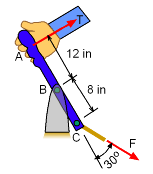Handbrake Mechanism Example A handbrake is designed to pivot about point B while connected to control rod at C as shown in the diagram on the left. What is the maximum force, T, that can be applied at A if the shear stress in the pin at B and axial stress in the control rod at C is limited to 12.5 ksi and 5 ksi, respectively? Assume the diameter of the circular rod and the pin is 1 in and 0.5 in, respectively. Solution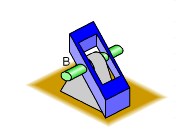Close-up of Pin Joint at B. Pin is Connect on Both Sides.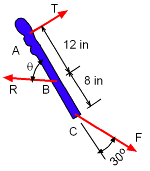Free-Body Diagram The applied force T may cause either shear failure in the pin at B or axial failure in the control rod at C. Shear failure will occur when shear stress in the pin at B exceeds 12.5 ksi. Since this stress is applied on two sides of same cross-section of the pin, the maximum allowable shear force is     Rmax = 2 [ 12.5 π (0.252) ] = 4.909 kip Axial failure will occur when axial stress in the control rod at C exceeds 5 ksi. The maximum allowable axial force in the control rod is      Fmax = 5 π (0.52) = 3.927 kip For convenience, it is assumed that the pin at B will fail in shear stress before the rod at C will fail in axial stress. The axial force, F, at this stress state will be determined and compared with Fmax . If F is less than or equal to Fmax, the assumption is correct. Otherwise the failure will occur due to axial stress and the value of T will have to be recalculated. Since it is assumed the pin at B will fail first, the force at B is set at 4.909 kip at an angle of θ, as shown at the left. Notice the x-y coordinates are orientated in the handbrake direction. There are three unknowns, F, T and θ.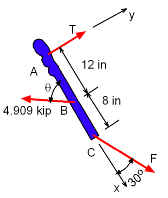Shear Failure Assumed Since it is assumed the pin at B will fail first, the force at B is set at 4.909 kip with an unknown angle of θ, as shown at the left. Notice the x-y coordinates are orientated in the handbrake direction. There are three unknowns, F, T and θ. Summing all forces in x direction, ΣFx = 0, gives      F cos30 = 4.909 cosθ      F = 5.669 cosθ Summing all forces in y direction, ΣFy = 0, gives      T = 4.909 sinθ - F sin30      T = 4.909 sinθ - 0.5 F Summing all moments about B, ΣMB = 0, gives      (T)(12) = (Fsin30)(8)      T = 0.3333 F Combining last two equations,      0.3333 F = 4,909 sinθ - 0.5 F      F = 5.891 sinθ Replacing F in the first equation,      5.669 cosθ = 5.891 sinθ      θ = tan-1(5.669 / 5.891)         = 43.90° Calculating for axial force,      F = 5,891 sin43.90         = 4085 lb > Fmax Hence, the assumption for failure is not correct and it is obvious that the failure will occur due to axial stress.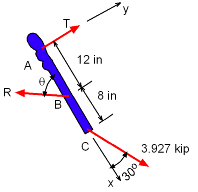Rod Tension Failure Assumed Since the control rod at C will fail first, the force at C is now set at 3.927 kip. There are three unknowns, R, T and θ. To determine the value of T, calculations with other two unknowns can be easily avoided by taking summation of all moments about B. ΣMB = 0, gives,      (T)(12) = (Fsin30)(8)      (T)(12) = (3.927sin30)(8)      T = 1,309 lb

Practice Homework and Test problems now available in the 'Eng Mechanics' mobile app
Includes over 400 problems with complete detailed solutions.
Available now at the Google Play Store and Apple App Store.